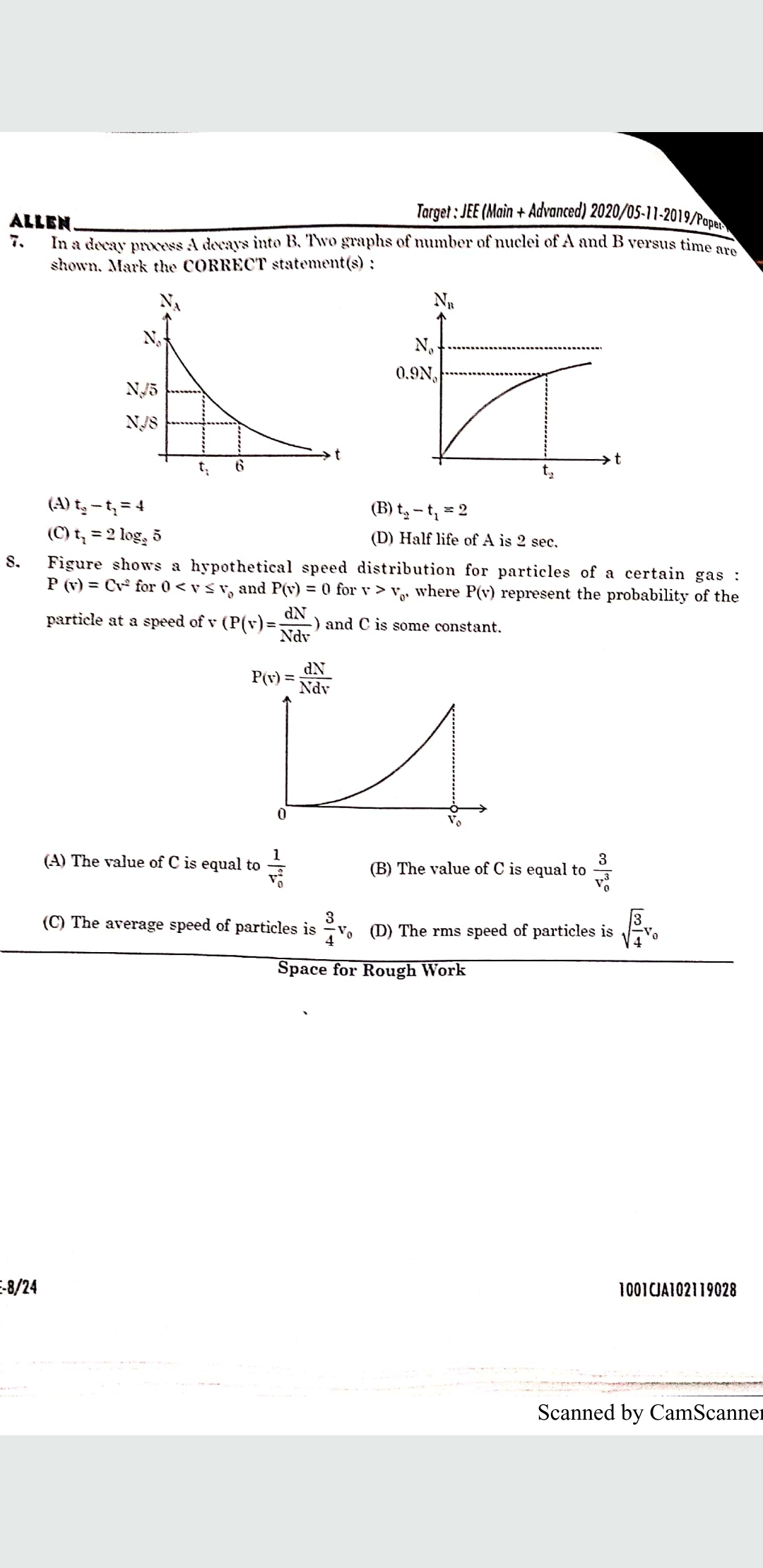# Doubt from KTOGQns 8

1 Like

1 Like

@akash_2020
Since total probability =1 or because P(v)dv can be thought of as representing the fraction of particles with velocity between v and v+dv, the following integral would represent the total fraction \cfrac{N_0}{N_0}= 1
\displaystyle \int_\limits0 ^\infty P(v)dv = 1
\displaystyle \int_\limits0 ^{v_0} Cv^2dv = 1
\Rightarrow C = \cfrac{3}{v_0^3}
Average velocity = \langle v\rangle= \displaystyle \int_\limits0 ^\infty vP(v)dv
\Rightarrow \langle v\rangle = \displaystyle \int_\limits0 ^{v_0} Cv^3dv = C\cfrac{v_0^4}{4}
\Rightarrow \langle v\rangle= \cfrac{3v_0}{4}

Mean of squares = \langle v^2\rangle = \displaystyle \int_\limits0 ^\infty v^2P(v)dv
\langle v^2\rangle = \displaystyle \int_\limits0 ^{v_0} Cv^4dv = C\cfrac{v_0^5}{5} = \cfrac{3v_0^2}{5}
RMS Velocity = \displaystyle v_{rms} = \sqrt{\langle v^2\rangle} = \sqrt{\cfrac{3}{5}}v_0

3 Likes

Thanks @Viram_2019 sir

1 Like